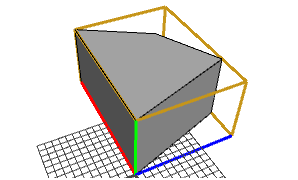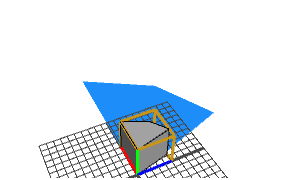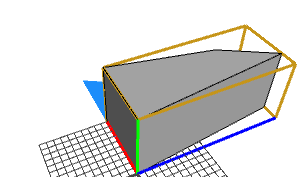s operation

Syntax

• s(xSize, ySize, zSize)

Parameters

1. xSizefloat
Size of the new scope for x-dimension.
2. ySizefloat
Size of the new scope for y-dimension.
3. zSizefloat
Size of the new scope for z-dimension.

Description

The s operation sets the size vector scope.s. The relative operator ' permits a convenient notation relative to the current shape's scope size:

s('sx,0,0) is equivalent to s(sx*scope.sx, 0, 0)

Negative sizes result in mirroring along the corresponding axes; this means the normals are inverted.

Examples

Basic Usage

 The initial shape with its scope highlighted.Lot--> extrude(10)Here, all scope sizes are set to the absolute value 5.Lot--> extrude(10) s(5,5,5)This example demonstrates the usage of the relative operator '. The s operation is equivalent to: s(0.5*scope.sx,scope.sy,1.5*scope.sz).Lot--> extrude(10) s('0.5,'1,'1.5)